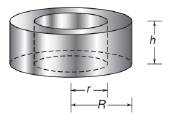Chapter 9.3, Problem 35EElementary Geometry For College St...

7th Edition
Alexander + 2 others
ISBN: 9781337614085

Solutions

Chapter
SectionElementary Geometry For College St...

7th Edition
Alexander + 2 others
ISBN: 9781337614085
Textbook Problem

In Exercises 34 and 35, give a paragraph proof for each claim.The volume V of a washer that has an inside radius of length r , an outside radius of length R , and an altitude of measure h is given by V = π h ( R + r ) ( R − r ) .To determine

To give:

The paragraph proof for the claim “The volume V of a washer that has an inside radius of length r, an outside radius of length R, and an altitude of measure h is given by V=πh(R+r)(Rr)”.

Explanation

Given:

The claim is “The volume V of a washer that has an inside radius of length r, an outside radius of length R, and an altitude of measure h is given by V=πh(R+r)(Rr)”.

Proof:

Consider a circular cylinder.

Let r be the inside radius, R be the outside radius and h be the altitude of the circular cylinder.

The volume of the circular cylinder with radius r is V1=πr2h

The volume of the circular cylinder with radius R is V2=πR2h

Hence the volume of the washer is V=V2V1

Still sussing out bartleby?

Check out a sample textbook solution.

See a sample solution

The Solution to Your Study Problems

Bartleby provides explanations to thousands of textbook problems written by our experts, many with advanced degrees!

Get Started

In Exercises 69-74, rationalize the numerator. 73. x2z3y

Applied Calculus for the Managerial, Life, and Social Sciences: A Brief Approach

Find the value of the sum. 22. i=36i(i+2)

Single Variable Calculus: Early Transcendentals, Volume I

In Problems 7-34, perform the indicated operations and simplify. 8.

Mathematical Applications for the Management, Life, and Social Sciences

In Exercises 5-8, find the limit. limx0(x+x)2x2x

Calculus: An Applied Approach (MindTap Course List)